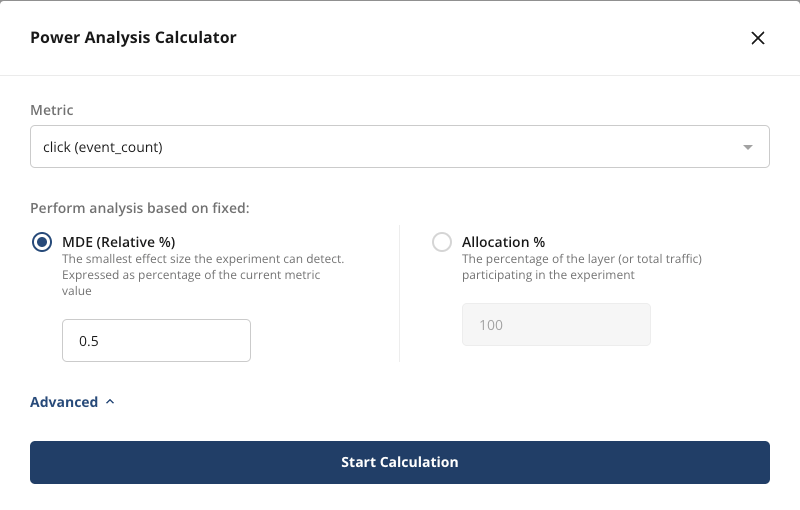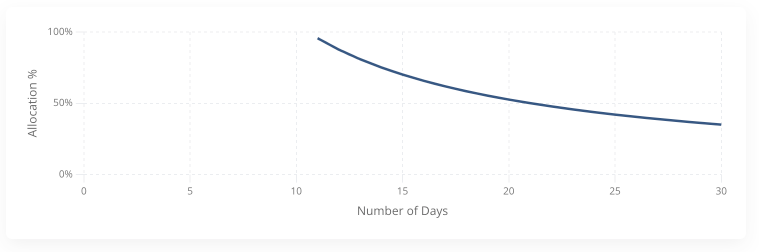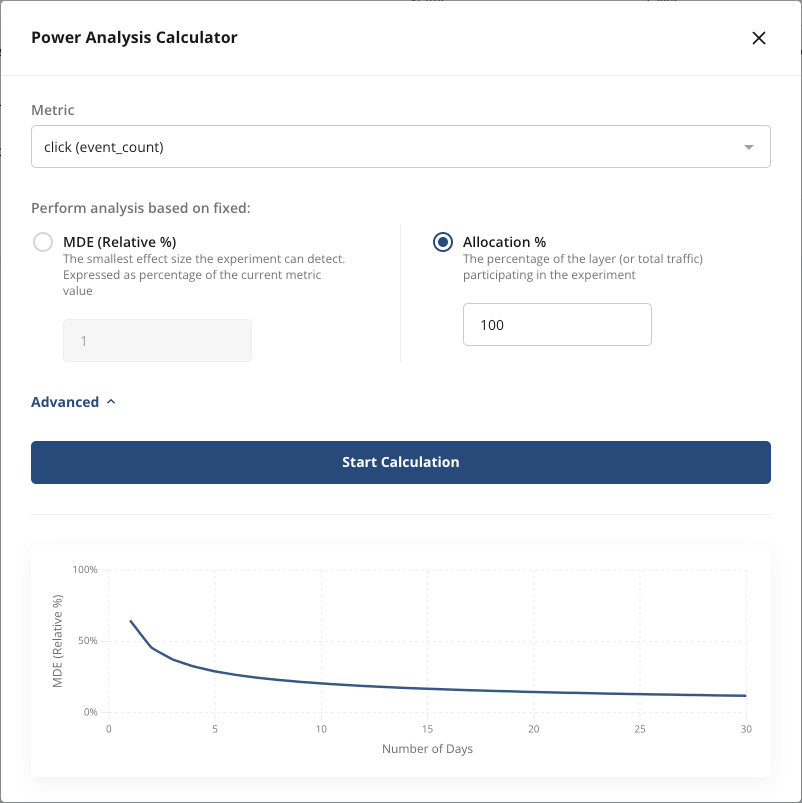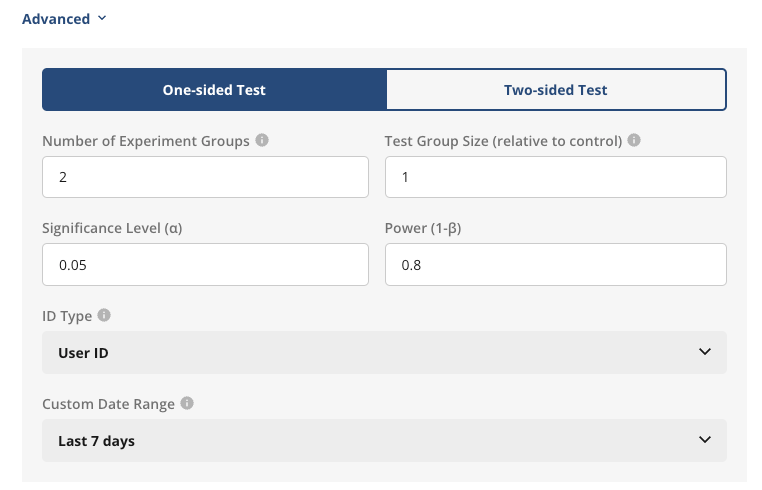# Power Analysis

The power analysis tool leverages past data for a given metric to estimate the relationship between three variables:

• Minimum detectable effect (MDE): The smallest change in the metric that the experiment can detect. For example: An MDE of 1% means that if there's a true effect of 1% or larger on our metric, we expect the experiment will show a statistically significant result. If the effect is smaller than 1%, then it will likely fall inside the confidence intervals and not be statistically significant.
• Number of days: How long the experiment is active. Longer running experiment typically have more observations, leading to tighter confidence intervals and smaller MDE.
• Allocation: The percentage of traffic that participates in the experiment. Larger allocation leads to smaller MDE, so it's often desirable to allocate as many users as possible to get faster or more sensitive results. When there's a risk of negative impact however, it's useful to know the smallest allocation that can achieve the desired MDE.

### Using the Tool​

To begin, launch the tool from the experiment creation flow:1. Select a metric of interest

2. Select the type of analysis to perform

3. Click on Start Calculation to see the results### Fixed MDE Analysis​

Choose this option if the smallest effect size that the experiment should detect is known. Enter the desired MDE as a percentage of the current metric value. For example: If a website currently gets 1,000 clicks per day, an MDE of 5% means we can detect a change of 50 or more clicks per day.

The results show the minimum number of days needed to reach this MDE for different allocation percentages. In the example below, the experiment should run for at least 11 days with 100% allocation or 21 days at 50%.### Fixed Allocation Analysis​

Choose this option to understand how the length of the experiment impacts the MDE. The example below shows how the MDE for a click count metric shrinks over time in an experiment with 100% allocation. On the first day the MDE is 64%, after 30 days it's down to 11%.